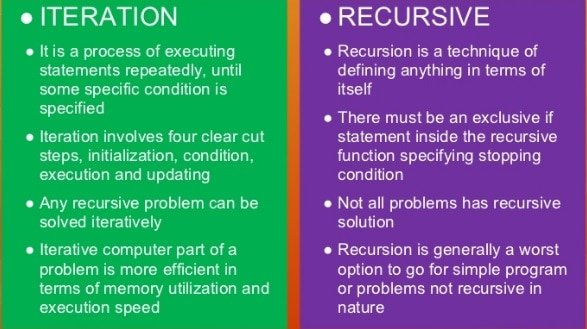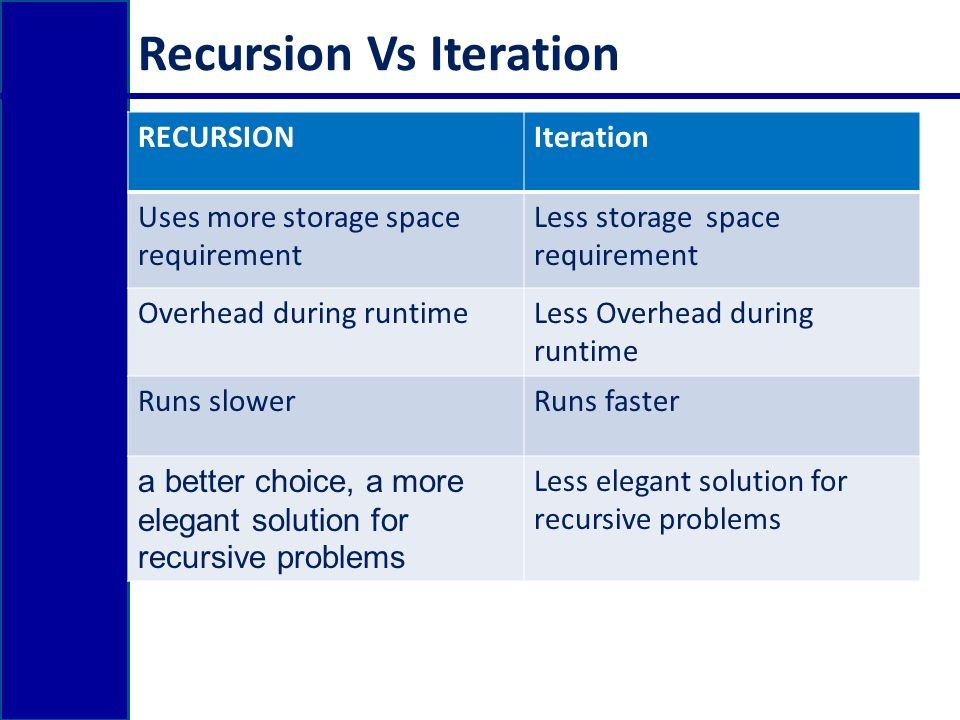# Difference between recursion and iteration

In programming languages like Java, C, C++, Python etc. whenever we want to call a function or a specific piece of code for several number of times or if we want to implement a function until a base condition is reached we use a procedure named as iterative process or we can also call a function again and again which is called as recursive function.In this article we will have a thorough discussion about the purpose usage and functionality of recursion and iteration and how they differ from each other and the do’s and don’ts while working with recursion and iterations. Lets’ start our discussion with recursion.

## Introduction to Recursion

The origin of word recursion lies somewhere between re occurrence, as is obvious from its name the tern is used when we want an event to happen several times (until our demand isn’t fulfilled). In programming languages like C, C++ and Java recursion is used with functions. Calling a function inside itself is called as recursion. But the question arise here is why we would need to call a function inside its definition? Whenever we used calculations where a same function needs to be executed again and again, we don’t write the program again instead we define a function for that process and call it whenever we want its’ execution again rather than writing the whole code again and again.

The procedure of calling a function inside its definition is also called as circular definition. However, the variables declared in that function will be instantiated again (at every call of the function) and the return address of the program counter along with the initialized variables will be stored to stack.

Functions like factorial and Fibonacci series always use recursion for their implementation because without recursion is not possible to write a general code which is working for all the cases. We will also work on an example of using recursion in this tutorial.

Size of the code:

The size of the code is reduced by using recursion as we don’t need to write the function again and again else, we just call the function several times. However, the size does not reduce significantly.

Termination condition:

The termination condition of recursive functions is a base condition which returns the value generated so far to the main code. Not understanding? You will when I will show you a piece of recursive function soon in an example below just go with the flow.

Example:

1. int factorial (int num) {
3. if (num==1) { //base condition
4. return 1;
5. } else {
6. answer = factorial(num-1) * num; //recursive calling converging towards num ==1
7. }
9. }

Infinite loop:

The infinite loop in recursion will cause the system to crash and this will happen when the recursive call does not converge towards the base condition. Hence the system will go on calling itself recursively without leading towards any termination. You might be wondering why? Lets’ conclude this for the time, let’s suppose our function do not have any base condition as given in the template below,

1. int factorial(int num){
3. answer = factorial(num-1) * num; //recursive calling
5. }

This will store the values of answer and the return address in stack at every recursive call of the function. But when will this end? NEVER. This will keep on saving the return address of the function call and the instance variables forever hence at a time our stack will overflow and crash the system, this is why base case of recursive function is very important. In the first template the else part is used for recursive call and the if part is the base condition.  Lets’ now discuss iteration and compare it with the recursive function call.

## introduction to Iteration

As we have seen in previous tutorial the difference between while and do while loop, at this point I am expecting that you know the working of loops and why we need looping. Every time a loop is called the control go to the conditional statement of loop, check whether it Is true or not. If it is true, the control will implement the statements inside the loop else it will skip the implementation and move to the next step after the loop. As we have seen the uses of recursion lets discuss why we use loops? A loop is used to implement a statement multiple times for instance if we want to print a statement 10 times, I will run a loop with a condition of 10 iterations instead of typing the print statement 10 times. Each time a loop is called back we call it a single iteration, now you must have a clear concept of iterations in loops. We have discussed this in detail in previous articles hence I am skipping further details this time.

Size of code:

The size of code having loops is not reduced much as compared to the recursive function because at the end the entire block is running over and over not effecting the entire size of the code.

Example:

1. int factorial (int num) {
2. int answer=1; //needs initialization because it may contain a garbage value before its initialization
3. for (int t =1; t>num; t++) //iteration
4. {
7. }
8. }

Termination condition:

The termination condition of a loop is the increment/decrement of the conditional variable. For instance, in the above code the variable t inside the for loop is the conditional variable the condition t++ inside the for-loop statement converges the loop to termination (or base condition which is t>num).

Infinite loop:

The loop will end up executing infinite times when the increment/decrement in the conditional variable do not converge. For instance, in the above case if the conditional variable decrements every time instead of increment i.e. t– in this scenario t will never become greater than num if num is a positive num and the condition statement will never turn false. This infinite loop will only run the code infinite times but do not crash the system as with the case with recursion.

Below is given the briefing of comparison between recursion and iteration.## Difference between with comparison table

Recursion Iteration
Definition Calling a function again and again Calling a specific piece/block of code again and again
Format A function calls itself unless a base case is achieved A block is called as long as a condition is true
Speed It is a very slow process due to involvement of stack Process is faster compared to recursion because stack is not involved
Termination Recursion terminated when base condition is achieved Iteration stops when conditional statement turns false
Infinite loop Infinite recursion will crash the complete system, because stack will be full after some time Infinite recursion will only execute the statement infinite time, but system will not crash
Size of code Size of code is reduced by recursion Size of code is larger while working with iterations
Stack At each recursion occurrence stack is provided an entry with return address Stack is not used in iterative processes
Usability Recursion can only be used with functions, by calling a function inside itself. Iteration can only be implemented by using loops only.